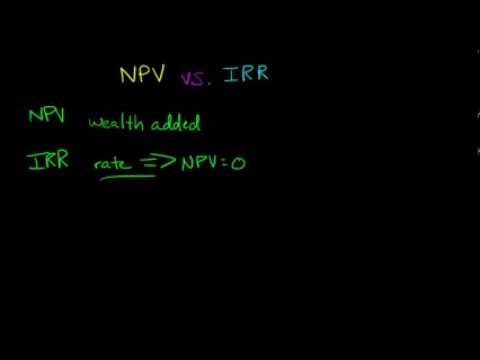### Main Difference

NPV stands for “Net Present Value” and IRR stands for “Internal Rate of Return”. Both the NPV and IRR are the two tools used for estimating the cost of any new project. The higher the values of these two parameters, the investment is considered to be more profitable. These tools are used to judge either the new project is favorable or profitable to launch or not. These two parameters are different from each other. Expression of both terms is different from each other. IRR is expressed in percentage while NPV is expressed in terms of currency. IRR is used for evaluating the projects with changing cash flows but NPV is unable to evaluate the changing cash flows projects. AS IRR method represents in percentage, so it is much more understandable by the manager with concepts of return.

### What is IRR?

IRR stands for “Internal Rate of Return” which is a tool used for capital budgeting. Capital budgeting is a process to evaluate the feasibility of the return on investment. It judges either the investment on a project will generate the expected profits or not. The proposed project should not be launched unless the value of IRR is positive. The higher value of IRR

### What is NPV?

NPV stands for “Net Present Value”. It is a tool to calculate the difference between cash inflow and cash outflow of organization at present time. Probability is found byt NPV. It gives the estimated value estimated cost of any project at present time and after few years the cost of same project keeping in view the inflation rate and others factors. It is helpful to buy any company or organization.

### Key Differences

1. Expression of both terms is different from each other. IRR is expressed in percentage while NPV is expressed in terms of currency.
2. NPV stands for “Net Present Value” While IRR stands for “Internal Rate of Return”.
3. NPV is preferred over IRR as NPV calculates additional wealth.
4. NPV is capable to calculate the additional wealth while IRR does not.
5. IRR is used for evaluating the projects with changing cash flows but NPV is unable to evaluate the changing cash flows projects.
6. AS IRR method represents in percentage, so it is much more understandable by the manager with concepts of return.
7. While using NPV, you will found different recommendations when using different discount rates but for IRR the recommendations are always the same.
8. Decision making is quite easy by using NPV while IRR does not help for decision making.
9. NPV determines the surplus from the project while IRR determines the state of Break Even Point.